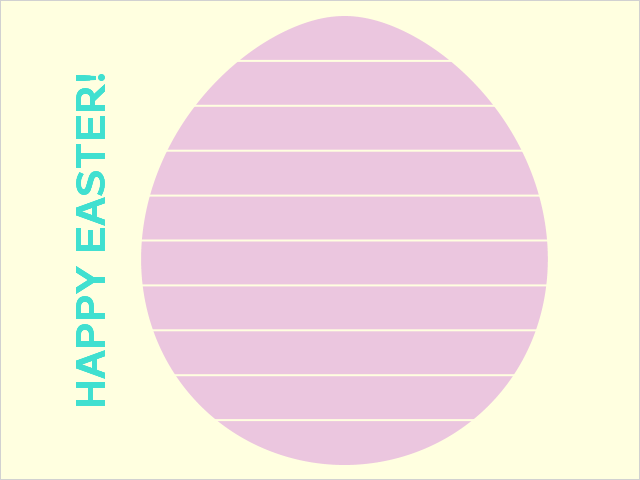## Fun With SAS ODS Graphics: Easter Egg Equation HuntSAS CODE

``````* Fun With SAS ODS Graphics: Easter Egg Equation Hunt

References
1. What is mathematical equation for an egg? (Quora)
https://www.quora.com/What-is-mathematical-equation-for-an-egg
2. Equation of Egg Shaped Curve of the Actual Egg is Found (Nobuo Yamamoto)
http://www.geocities.jp/nyjp07/index_egg_E.html
3. Solution for (x^2+y^2)^2 = x^3+(3/10)xy^2 (Wolfram)
http://www.wolframalpha.com/input/?i=(x%5E2%2By%5E2)%5E2+%3D+x%5E3%2B(3%2F10)xy%5E2
4. The Colors of Easter
https://www.lifewire.com/the-colors-of-easter-4126619;

data egg;                                    * Use formula to create "Easter egg" polynomial points;
id=1;
do x=0 to 1 by .0001;
y = sqrt(-20*x**2 + sqrt(280*x + 9)*x + 3*x)/(2*sqrt(5));
output;
end;
do x=1 to 0 by -.0001;
y = -sqrt(-20*x**2 + sqrt(280*x + 9)*x + 3*x)/(2*sqrt(5));
output;
end;

ods graphics / antialias ANTIALIASMAX=20100; * Polygon plot of "Easter egg";
proc sgplot data=egg aspect=1 nowall noborder;
styleattrs backcolor=cxffffe0;
polygon x=y y=x id=id / nooutline fill fillattrs=(color=cxdda0dd) transparency=.4; * Change orientation (x=y, y=x);
yaxis reverse display=(noline noticks novalues) label="HAPPY EASTER!" labelattrs=(size=30pt color=cx40e0D0 weight=bold);
xaxis display=none;
refline .1 to .9 by .1 / lineattrs=(color=cxffffe0 thickness=2pt) axis=y; * Easter egg "stripes";
run;``````
3 REPLIES 3Jay54
Meteorite | Level 14

## Re: Fun With SAS ODS Graphics: Easter Egg Equation Hunt

Awesome!Jay54
Meteorite | Level 14

Awesome!

## Re: Fun With SAS ODS Graphics: Easter Egg Equation Hunt

awesome...loved it.

Discussion stats
• 3 replies
• 1600 views
• 9 likes
• 3 in conversation